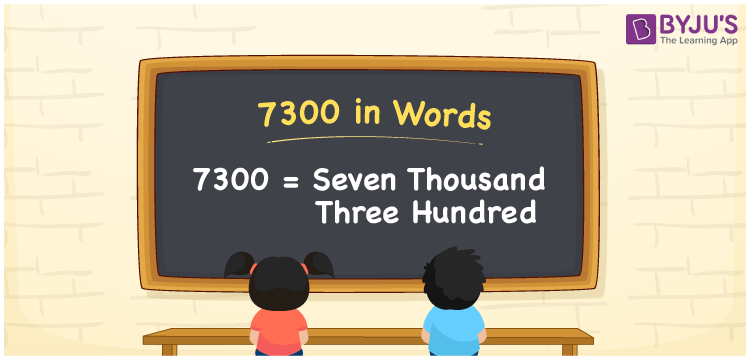# 7300 in Words

7300 in words can be written as Seven Thousand Three Hundred. You will learn the concept of numbers in words effectively in this article. If you spend Rs. 7300 at the restaurant, then you can say that “I spent Seven Thousand Three Hundred Rupees at the restaurant”. So 7300 can be read as “Seven Thousand Three Hundred” in words.

 7300 in words Seven Thousand Three Hundred Seven Thousand Three Hundred in Numbers 7300

## 7300 in English Words## How to Write 7300 in Words?

In this section, the place value of 7300 is explained in a clear cut manner. The place value chart of each digit in the number 7300 is shown in the table below.

 Thousands Hundreds Tens Ones 7 3 0 0

7300 in expanded form can be written as:

7 x Thousand + 3 × Hundred + 0 × Ten + 0 × One

= 7 x 1000 + 3 × 100 + 0 × 10 + 0 × 1

= 7000 + 300

= 7300

= Seven Thousand Three Hundred

Hence, 7300 in words is written as Seven Thousand Three Hundred.

7300 is a natural number that precedes 7301 and succeeds 7299.

7300 in words – Seven Thousand Three Hundred

Is 7300 an odd number? – No

Is 7300 an even number? – Yes

Is 7300 a perfect square number? – No

Is 7300 a perfect cube number? – No

Is 7300 a prime number? – No

Is 7300 a composite number? – Yes

## Frequently Asked Questions on 7300 in Words

Q1

### How to write 7300 in words?

7300 can be written in words as “Seven Thousand Three Hundred”.
Q2

### Write Seven Thousand Three Hundred in numbers.

Seven Thousand Three Hundred in numbers can be written as 7300.
Q3

### Is 7300 an even number?

7300 is an even number as it is completely divisible by 2.